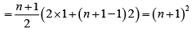Courses

# Test: Binomial Theorem (Competition Level)- 3

## 30 Questions MCQ Test Mathematics For JEE | Test: Binomial Theorem (Competition Level)- 3

Description
This mock test of Test: Binomial Theorem (Competition Level)- 3 for JEE helps you for every JEE entrance exam. This contains 30 Multiple Choice Questions for JEE Test: Binomial Theorem (Competition Level)- 3 (mcq) to study with solutions a complete question bank. The solved questions answers in this Test: Binomial Theorem (Competition Level)- 3 quiz give you a good mix of easy questions and tough questions. JEE students definitely take this Test: Binomial Theorem (Competition Level)- 3 exercise for a better result in the exam. You can find other Test: Binomial Theorem (Competition Level)- 3 extra questions, long questions & short questions for JEE on EduRev as well by searching above.
QUESTION: 1

### Coefficient of x5 in the expansion of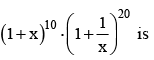Solution:

Coefficient of xin (1 + x)10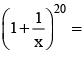Coefficient of x25 in (1 + x)30

QUESTION: 2

### The coefficients of 9th, 10th and 11th terms in the expansion of (1 + x)n are in A.P. then n =

Solution:

(n - 2r)2 = n + 2

QUESTION: 3

### Coefficient of x4 in (1 + x – 2x2)6 is

Solution:

Factorise and expand

QUESTION: 4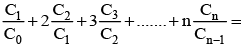Solution:

Consider the given function:
c1/c0 + 2c2/c1 +  3c3/c2 + 4c4/c3 +......+ncn/cn−1
=n/1 + [2n(n−1)]/2! . 1/n + [3n(n−1)(n−2)]/3! . 1/[n(n−1)/2!] +......+n.1/n
=n+(n−1)+(n−2)+......1
=1+2+3+......+n
= n(n+1)/2

QUESTION: 5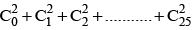= (where nCr = 25Cr)

Solution: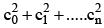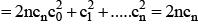QUESTION: 6

It is suitable to use Binomial Distribution only for

Solution:

As the value of ‘n’ increases, it becomes difficult and tedious to calculate the value of nCx.

QUESTION: 7

The number of terms in the expansion of [(a + 4b)3 + (a - 4b)3]2 are

Solution:

[2(a3 + 3.a.162)]2

QUESTION: 8

If (1+x+x2)n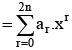then a1 - 2a2 + 3a3 - ... - 2na2n = ....

Solution:
QUESTION: 9

The sum of the binomial coefficients of the 3rd, 4th terms from the beginning and from the end of (a + x)n is 440 then n =

Solution:

Applying the above conditions, we get
nC2 + nC3+ nCn−2 + nCn−3 = 440
2(nC2 + nC3)=440
nC2 ​ + nC3=220
n(n−1)/2!+ [n(n−2)(n−1)]/3!=220
n(n−1)/2 ​(1+(n−2)/3=220
n(n−1)/2 (n+1)/3=220
n(n2−1)/6 ​= 220
n3−n=1320
n3−n−1320=0
The only real root of the above equation is 11.
Hence n=11

QUESTION: 10

Let R = (5√5 + 11)2n+1, f = R - [R], then Rf =

Solution:

Given, G = (5√5 + 11)2n + 1 , 0 < G < 1 and R = (5√5 + 11)2n + 1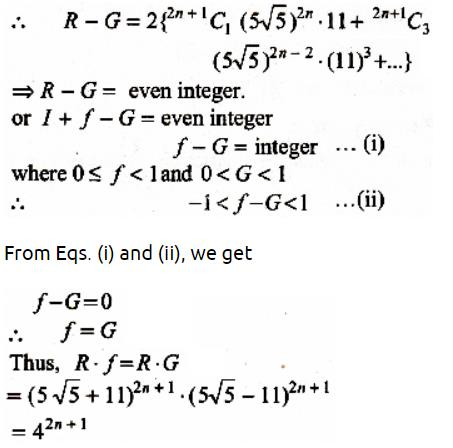QUESTION: 11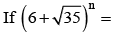I + F when I is  odd and 0 < F < 1, then (I + F) (I - F) =

Solution: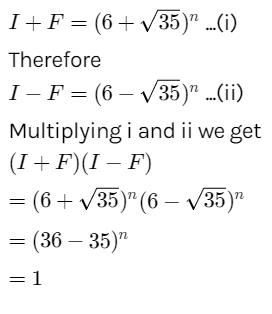QUESTION: 12

The expansion [x + (x3 - 1)1/2]5 + [x + (x3 - 1)1/2]is a polynomial of degree

Solution:

Expand using the formula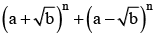QUESTION: 13

If t0, t1, t2, ............tn are the consecutive terms in the expansion (x + a)n then (t0 - t2 + t4 - t6 + ....)2 + (t1 - t3 + t5....)2

Solution:

Expand (x + ai)n and (x – ai)n then multiply.

QUESTION: 14

Coefficient of x50 in (1 + x)1000 + 2x(1 + x)999 + 3x2 (1 +x)998 +....is

Solution:

Take (1 + x)1000 as common, after simplification it becomes (1 + x)1000 coefficient of x50 is 1002C50

QUESTION: 15

The coefficient of x9 in  (x + 2) (x + 4) (x + 8).....(x + 1024) is

Solution:

2 + 4 + 8 + …..1024

QUESTION: 16

The coefficient of xn in the polynomial (x +n C0) (x + 3.nC1) (x + 5.nC2)...[x + (2n+ 1).nCn] is

Solution:

Coefficient of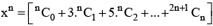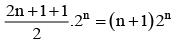QUESTION: 17

If x = (2 +√3)n, n ∈ N and f = x - [x], then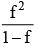Solution:

Explanation : x = (2 + (3)1/2)n

For n = 1

x = 2 + (3)1/2

f = x - [x]

=> [2 + (3)1/2] - [2 + (3)1/2

=> (3)1/2 - 1

f2 = [(3)1/2 - 1]2

=> 2(2 - (3)1/2)

1 - f = 1 - ((3)1/2 - 1)

1 - f = (2 - (3)1/2)

f2/(1-f) = [2(2-(3)1/2)]/[(2-(3)1/2)]

For n = 2

f = (7 + 4(3)1/2) - (7 + 4(3)1/2)

f = 7 + 4(3)1/2 - 13

f = 4(3)^1/2 - 6

f2 = [4(3)1/2 - 6]1/2

=> 48 + 36 - 48(3)1/2

=> 84 - 48(3)1/2

1 - f = 1 - (48(3)1/2 - 6)

1 - f = 7 - 4(3)1/2

f2/(1-f) = [84 - 48(3)1/2]/[7 - 4(3)1/2]

= 12(Even)

QUESTION: 18

If 22006 - 2006 divided by 7, the remainder is

Solution:

22006 = 4(8)668 = 4 (7 + 1)688 leaves remainder 4
2006 = 7 x 268 + 4 leaves remainder
∴ 22006 - 2006 leaves remainder

QUESTION: 19

In a Binomial Distribution, if p, q and n are probability of success, failure and number of trials respectively then variance is given by

Solution:

For a discrete probability function, the variance is given by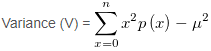Where µ is the mean, substitute P(x)=nCx px q(n-x) in the above equation and put µ = np to obtain
V = npq

QUESTION: 20

The coefficient of the term independent of x in the expansion of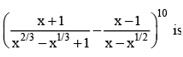Solution: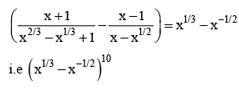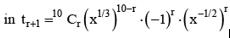for this term to be independent of x. we must have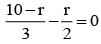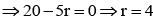So, the required coefficient is

10C4(-1)4 = 210

QUESTION: 21

The coefficient of x12 in the expansion of (1+2x2 - x3)8

Solution:
QUESTION: 22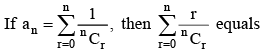Solution:
QUESTION: 23

The value of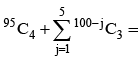Solution:

95C4 + 95C3 + 96C3 + 97C3 + 98C3 + 98C4 + 99C3
= 96C4 + 96C3 + 97C3 + 98C3 + 99C3
= 97C4 + 97C3 + 98C3 + 99C3
= 98C4 + 98C3 + 99C3 =  99C4 + 99C3 = 100C4

QUESTION: 24

Coefficient of x11 in the expansion of (1+x2)4 (1+x3)7 (1+x4)12 is

Solution:
QUESTION: 25

The number of irrational terms in the expansion of (21/5 +31/10)55 is

Solution:

(21/5 +31/10)55 is
Total term = 55 + 1 = 56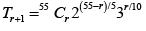Here r = 0, 10, 20, 30, 40, 50
Number of rational terms = 6;
Number of irrational terms = 56 - 6 = 50

QUESTION: 26

In the expansion of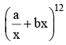, the coefficient of x-10 will be

Solution:

Given expansion is∴ General term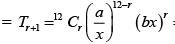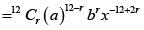Since, we have to find coefficient of x-10 ∴ -12 + 2r = -10  ⇒ r = 1
Now, then  coefficient  of x -10 is 12 C1(a)11 (b)1 = 12a11b

QUESTION: 27

The middle term in the expansion of (1 – 2x + x2)n is

Solution: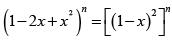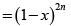Here 2n is even integer, therefore,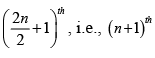term  will be  the middle term.
Now, (n + 1)th term in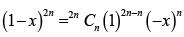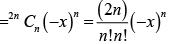QUESTION: 28

If (1 - x + 2x2)10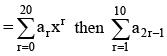equlas

Solution: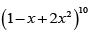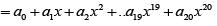Replacing x by -x,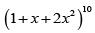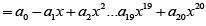Subtracting and putting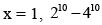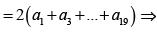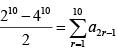QUESTION: 29

(103)86 - (86)103 - 86 is divisible by

Solution:

Given expression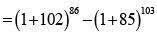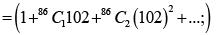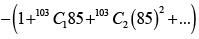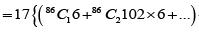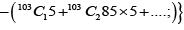QUESTION: 30

The number of dissimilar terms in the expansion of (a  + b + c)2n+1 – (a + b – c)2n+1 is

Solution:

Given expansion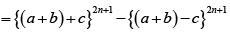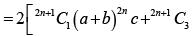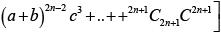Number of dissimilar  term = (2n + 1) + (2n -1) + ....(2n - 3) + .... + 5 + 3 + 1